## Sunday, December 26, 2010

Mayan calendar

UPDATE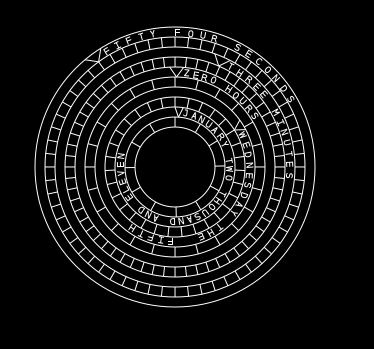I've just been cleaning up the code and found a couple of errors that have been corrected. You can find the code for the above here.

--Original post below-----------------------

I have been thinking about setting up a "year clock" using multiple rings showing progress in the year, month, day, hour, and minute. I had come up with some designs like so:In this case the largest circle is the year and the dot on the circle is where we are in the year. The next largest circle is the month and the dot where we are in the month and so on. I had set it up so that everything moved in increments of 1 second. The right side shows what the set up would look like on midnight of December 31st. The nice thing about this clock is that it will never look the same twice in a year. However, it isn't exactly easy to read :)

Next I tried this:Which uses similar mechanics as the clock above, but as you can see the rings are marked with information. Each circle rotated so that the current date/time was at the top of the ring. Unfortunately this clock proved to be extremely cpu intensive (it was actually pushing my cpu to 100%). I reoriented the circles to this: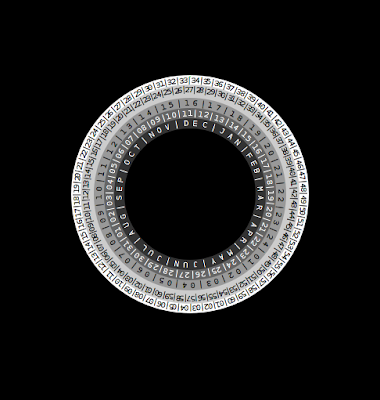But there was no was this script was going to be viable. But I liked the look of the above, with the rings within rings.

Then later I had the idea to try something else and this is what I came up with:This is when things started looking very Mayan :)
In the above calendar clock, year is the innermost circle and instead of having the circles move I had indicators move around the circles. I also went on to add some system info indicators like so: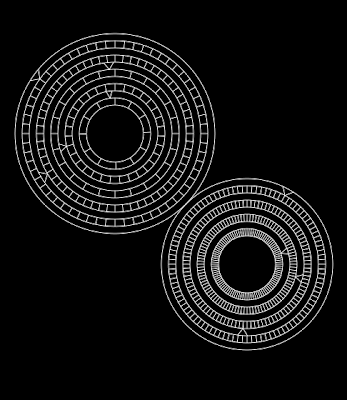The code for the above is here.

Sector11 has also made some cool looking additions to the calendar part. You can see that here. I have a nice Mayan symbol dingbat font that may well be used in conjunction with my circlewriting script to add some glyphs to the calendar also.
I posted a perspective bar set up some time ago, and thought I would revisit a 3D approach. This is what I ended up with.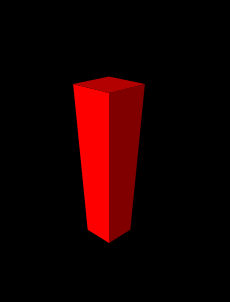The bar rises and falls in relation to whatever conky output you feed it. The script can be found here.

It took a crash refresher course in basic trigonometry to get everything working and I can't guarantee that if you try the script it will work. The bar looks as I wanted it to, but I was hoping to be able to define simple coordinates and have multiple bars generated that all fitted together.

This didn't work out in this script as I the math I was using wasn't calculating the right perspectives for subsequent bars.
Updates, I have a couple of things that I've been working on recently.

First there is a amalgamated circle generating and circlewriting lua script.
It can do things like this:And this is a little more complicated (obviously you can get whatever colors you want for rings and text I was just feeling monochrome when I made these.)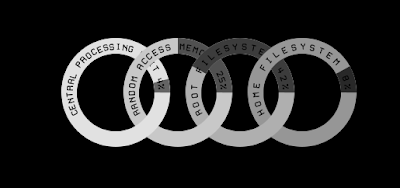The intertwining effect is made by making different bits of the circle at different points in the lua script. In lua the later instances of cairo graphics are drawn above any earlier instances.

The script can be found here on the crunchbang Linux forum (where you can see a more colorful use of the script. It can also generate bars that can be placed and rotated freely.

## Thursday, October 14, 2010

### Splitting text into individual letters

One Lua trick that I use a lot is taking a string and splitting it up into individual characters which then get stored in a table.

I used this approach for my circle writing function and many other functions. This is how it's done.

You need the following function in the Lua script above the main function. I did not write this function and I cant remember exactly where I got it from but whoever did write it has my thanks!

function string:split(delimiter)
local result = { }
local from = 1
local delim_from, delim_to = string.find( self, delimiter, from )
while delim_from do
table.insert( result, string.sub( self, from , delim_from-1 ) )
from = delim_to + 1
delim_from, delim_to = string.find( self, delimiter, from )
end
table.insert( result, string.sub( self, from ) )
return result
end

These are the lines that split up the text...
`text="text"print (text) --> textsub=string.gsub(text, ".", "%1|")-- the above inserts "|" after every characterprint (sub) --> t|e|x|t|split=string.split(sub, "|")-- the above splits the string whenever a "|" occurs and also deletes the "|"the resulting split up characters are stored in a table called split in this caseslen=string.len(text)-- slen is the length of the original text,so will be the number of entries in that table "split"so that:split = "t"split = "e"split = "x"split = "t"now I can deal with each character individually.I could give each character a different font, or a different coloror, in the case of my square font conky below, convert each character to the square font.`

### Square text conky

I had an idea for a conky. I wanted it to be extremely compact and involve text in different orientations. This is what I ended up with!The problem was that I spent a long time looking for a square font. I found several fonts that I liked but when I came to rotate them and put rotated letters next to regularly orientated letters I found that none of the fonts were actually square. Non were the same width as height.

So I decided to make my own font using the Lua script. I had done something similar in my ascii text lua; a function that read each letter of the text I wanted displayed and converted the letter into ascii.

In this case I created my own square font out of the webdings font. With webdings a "g" gave me a solid color square, while "c" gave me a black square with a color outline. I simply constructed my letters using those blocks like so:

`if letter=="T" thenfont="webdings"acrosst=acrossdownt=downrotate=rotate*math.pi/180cairo_translate (cr, acrosst, downt)cairo_rotate (cr, rotate)cairo_select_font_face (cr, font, CAIRO_FONT_SLANT_NORMAL, CAIRO_FONT_WEIGHT_NORMAL);cairo_set_font_size (cr, fontsize);cairo_set_source_rgba (cr, red, green, blue, alpha);cairo_move_to (cr, 0, 0);cairo_show_text (cr, "ggggg")ldown=fontsizecairo_move_to (cr, 0, ldown);cairo_show_text (cr, "ccgcc")ldown=fontsize+ldowncairo_move_to (cr, 0, ldown);cairo_show_text (cr, "ccgcc")ldown=fontsize+ldowncairo_move_to (cr, 0, ldown);cairo_show_text (cr, "ccgcc")ldown=fontsize+ldowncairo_move_to (cr, 0, ldown);cairo_show_text (cr, "ccgcc")cairo_rotate (cr, -1*rotate)cairo_translate (cr, -1*acrosst, -1*downt)end`
I actually really like the look of the resulting square "font". And everything fitted nicely together with no gaps :)

Go here to take a look at the code via the link. This is another really long script, mainly because of the letter conversion function. Originally I set up the function converting lowercase letters into upper case letters of the square font. But I didn't like the way they looked, so I re-wrote the letter conversion function to include converting uppercase letters to a different version of the square font.

I just used the lua command string.upper to make everything uppercase and fed it to the conversion function. I never bothered to delete the lowercase conversion lines :)

### Final Fantsy VII

I am a big final fantasy 7 fan, so when rabidofx posted a FF7 themed conky, I immediately thought of the potential to turn the FF7 bottom combat panel into a conky display.

And this is what I eventually came up with!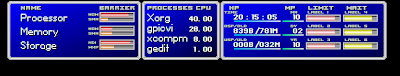I have tried to be as faithful as I can to the FF7 panel while still allowing the conky to function as a source of information.

It still needs some work and the script needs a good cleanup and improvements to make it more user friendly. You can get the code here at conky-pitstop! Or here on the crunchbanglinux forum. While your on the forum check out rabidfox's post directly above. He has modified ADcomps Adeskbar to look like materia slots (very nice!).

So why does it take 2200 lines of lua code to produce my FF7 panel conky?
Well 3 reasons... first because it's just a little messy. A lot of copying and pasting went on and I'm sure that I could comb through it an eliminate a large number of supurflouous lines :)

Second drawing in lua with the cairo library takes a lot of lines. To draw the blue shaded rectangles required this:
``sa=5sd=5rh=120rw=280red=1green=1blue=1alpha=1pat = cairo_pattern_create_linear (sa, sd, rh, rw)cairo_pattern_add_color_stop_rgba (pat, 0, red-1, green-1, blue, alpha)cairo_pattern_add_color_stop_rgba (pat, 1, red-1, green-1, blue-1, alpha)cairo_set_source (cr, pat)cairo_rectangle (cr, sa, sd, rw, rh)cairo_fill (cr)``
I could have used less lines and specified the positions and colors etc in the actual pattern and drawing lines, but this way is just so much easier for fine tuning. I set the colors up like this becasue I kept forgetting which number represented what in the cairo_set_pattern lines! :)

The grey border around the blue was also line intensive.

Reason 3, and perhaps the main reason for the length of the script is the way you have to use cairo to display text. And if you look closely you will see that every piece of text has a black "shadow" ...which is simply the same piece of text, colored black and printed behind and offset a little.

``font="Acknowledge TT (BRK)"text=topl1fsize=20red=0green=0blue=0alpha=1across=32down=22cairo_select_font_face (cr, font, CAIRO_FONT_SLANT_NORMAL, CAIRO_FONT_WEIGHT_NORMAL);cairo_set_font_size (cr, fsize);cairo_set_source_rgba (cr, red, green, blue, alpha);cairo_move_to (cr, across, down);cairo_show_text (cr, text)red=0.8green=0.8blue=0.8alpha=1across=across-2down=down-2cairo_set_source_rgba (cr, red, green, blue, alpha);cairo_move_to (cr, across, down);cairo_show_text (cr, text)``
So I had to set the font, set the font size, set the red, green, blue and alpha, set the position, write the font, fontsize and color setup lines, move cairo to the coordinates and then tell it to diaplay the text. That gave me the shadow, then I had to change the color and position, set up the text drawing variables and draw the main text.

I kept this format throughout the script, so i could easily change colors and positions without wading through a sea of setup lines.

## Saturday, September 11, 2010

Here is my latest conky configuration

conkybar!

and this is what it looks like currently: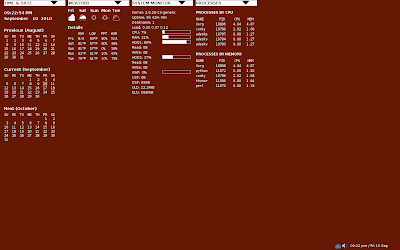Nothing out of the ordinary in terms of content over many other conkyrc generated displays.

But with a few differences.

The first thing is that every is generated in a lua script. The second is that those black triangles at the top are clickable buttons. Here is how this setup functions.

A while ago on the crunchbanglinux website a poster by the name of jpope had a wallpaper that had a large power button right in the middle of the screen. Here is the post:
http://crunchbanglinux.org/forums/post/45907/#p45907

ADcomp had written a python script to place a button on the desktop that would launch a command or script. The button could be placed anywhere and you could make your own icons for it to use and it could execute any command when you click it. It was all set up in the script.

I thought that was pretty cool and I stored it in the back of my mind.

Then recently I started looking into the file io function of lua, the ability to open files in the lua script, read their contents and store that as strings which could be manipulated in the script. I had been working on some bash scripts using curl to download information from webpages, then editing the html to pull out specific pieces of information.

I had been using the conky_parse command in lua to execute the bash scripts via conky, but I was trying to bring some of the operations into the native lua language to make the scripts run a little lighter cpu wise.

However, once I started playing around with the file io commands I realised that I could affect the whole lua script by having the script "watching" a text file and then editing the file, initially via a terminal command.

something like this:
\$ touch /tmp/setw.txt; echo "1" > /tmp/setw.txt

the touch command creates the file then the > writes a "1" into the file.
a similar command could then easily ovewrite the "1" with a "2"

so if i had the Lua script watching that file I could put the output into a string and use the string as a basis for an if statement.

These are the lines that watch the text files in the lua script

I've changed the names and moved the files out of /tmp so they are persistant.

So now a simple:

if wset=="1" then
--display one thing--
end

if wset=="2" then
--display something else--
end

gives me interactive control over the script.

take a look at this entry on the ubuntu conky thread for more infomation on how the interaction works.
http://ubuntuforums.org/showpost.php?p=9826304&postcount=13840

The actual lua script can be found here. It is still very much a work in progress.
http://ubuntuforums.org/showpost.php?p=9830734&postcount=13855

The fact that everything has to be constructed in lua was itself a challenge. Lua doesn't have all the goodies pre-programmed into it like the objects you can get in a conkyrc by putting in the right command

The calendars were tricky. I saw londonali1010's post on lua calendars here
http://mylittledesktop.blogspot.com/2009/10/argh-my-first-snag-with-my-lovely.html

I looked over her code and borrowed a couple of lines (for calculating start day), but went about contructing the calendar in a very different way. The result was a calendar that looked very similar to the one available through a conkyrc. I'm still trying to perfect the day indicator however.

The biggest limitation to using Lua to construct a complete conky output is how it handles displaying text. It takes a lot of lines to get the tables you see in my output, far more than if I were to do the same thing in a straight conkyrc.

Anyway, I'm still working on the content of the conky but I am happy how the mechanics have worked out!

As it stands right now this setup is no more cpu draining than my regular all conkyrc setup. And when I have all the sections rolled up (except system monitor so that I can see the cpu usage) it runs much lighter. When a section is "rolled up" or inactive the lua script does not have to do any of the calculations or rendering for that section.

## Sunday, August 29, 2010

Animated text conky.
Here is a concept for a conky that I have been thinking about for a while.

having the text in conky appear as if it were being typed.

The idea came a while back when I was thinking about how to get a better scroll function in conky, and I was working on a matrix rain themed conky. I thought it would be cool if you could get text in conky to appear like the text that appeared on Neo's computer in the movie.

Thanks go to iggykoopa of the crunchbanglinux forum for his help with coding. Other wise the script would be very difficult to change. Now you can set up the script to display any text you like, when you like and where you like. It's still a bit tricky as you have to work out your timings for each section of text to appear, pause and vanish.

I have set it up so that you get the impression that a program is runing inside of conky, scanning your system and fetching the information.

You can get the code on the crunchbang linux forum here.

## Saturday, July 31, 2010

My latest conky plots the position of the sun throughout the day. The Lua script also calculates and displays the sun's altitude and azimuth as it changes throughout the day. I had to get to grips with some equations and also get to grips with how to do those calculations in Lua.

The conky has 2 scripts that make it work.
Firstly there is a bash script that uses the curl function to access a webpage. Then using grep, awk and sed I extracted key astronomical data from the page.

The Lua script is then fed the data via conky and uses the numbers to perform all the necessary calculations. I also display a range of data in the conky via the Lua script.The figure is all based on equations which are in turn based on the data I gathered. The figure shows a very accurate representation of the position of the sun relative to the horizon.

You can get the code for the bash and Lua script on the crunchbang linux website here.

If you are interested in using this conky then be sure to read my post as you will have to make some changes to the scripts regarding geographical location.
Here is a new conky/lua setup from me.

This is based on a wallpaper created by the ever talented gutterslob which he posted on the crunchbang linux website here.

This was posted back in March and I said at the time that it would make a great conky but I never went any further than that. Then recently I saw someone else on the crunchbang site post a screenshot using the wallpaper and, as I had some time to kill, I started making a conky display based upon it.

This is how it turned out (everything is labeled in the picture)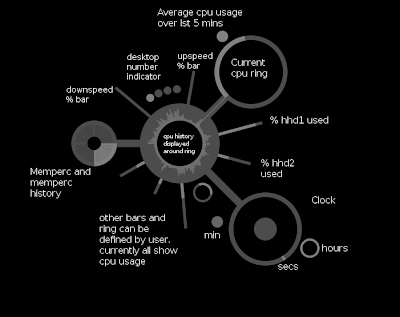The code is on the crunchbang website here.
The code is ugly, overlong, overcomplicated and far from user friendly. The Lua script is an amalgamation of bits of previous lua scripts with modifications but I have yet to go through the code and clean it up.

There are some innovations. I think my clock turned out quite nicely and I haven't seen a clock like that before. I also liked the idea of a wordless/numberless figure (which this is)

Also my upspeed and downspeed bars, while seemingly simple, display speed as a percentage of total possible speed (determined via speed testing). The lua script can differentiate between bytes, kilobytes and megabytes per second (that is output by the conky object upspeed for example) and calculate speed in a single unit which makes this possible.

## Tuesday, March 16, 2010

I haven't been very active conky wise lately and it looks like I won't be able to spend much time in the future on conky/Lua tinkering either.

I have projects that are going to be keeping me busy. But hopefully I will have a few hours here and there to play!

I've still got lots of good ideas about things to do with Lua/cairo scripts, but as the scripts get more complicated they also take longer to write and test.

I guess I'm entering a mostly retired state as far as conky goes.

## Saturday, March 6, 2010

Numbers to text conversion functions.
Code available on the crunchbanglinux forum here.I have written 3 functions for converting various numbers to text representations.
The first function converts any regular 3 digit or lower number into words, so that:
19 becomes "nineteen" and 487 becomes "four hundred and eighty seven"
I have used this function in the third line in the image above to convert the output of \$cpu to words.

The second function converts number dates into text, as in the first line in the image above.

The third converts time data to words as in the middle line above. This function takes into account all the changes that occur with displaying the time as words. For example at 6:01, the function will output "one minute past six". At 6:02 it will be "two minutes past six". At 6:00 it will show "six o'clock" etc. You can choose between having seconds represented or not.

I intend to use these functions with my circlewriting functions to create a text only, non-linear display along the lines of this: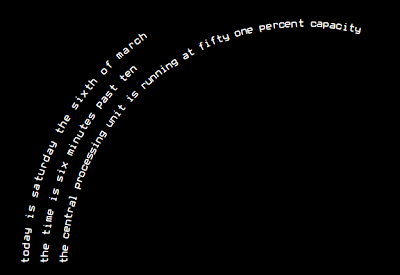here is what it looks like when its working:

## Monday, March 1, 2010

UPDATE. I have made the perspective drawing function configurable and posted the code on the crunchbanglinux website here. The code there displays the following graphic.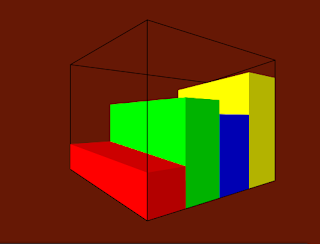Because everything is based off of equations (which are themselves based off just a few key pieces of data), you can easily use the settings to alter the look of the bars like so:

In this case the red bar is cpu usage.
The green is memperc.
The blue and yellow bars show the percent used of my hard disk drives.
There is a maximum of 6 bars assignable. For more bars the Lua script would have to altered to add them manually.

ORIGINAL POST

I have also been thinking more about creating more isometric figure drawing functions. I put together this one a little while ago. While it does look cool, it clearly does not reflect any kind of reality of how a 3D shape would look from a fixed perspective (which is what isometric means).

So I thought about trying to create a more realistic looking figure. I knew it would involve the use of perspective, but I had no idea about how to create the effect or the mathematical principles behind perspective.

So I turned to google and one of the first things I came across was this video.
That gave me a basic understanding of how the effect was achieved. But I didn't want to simply draw a figure using fixed coordinates (that would have been far too easy), I wanted to have a isometric figure that could be configured through settings.

I had to rapidly re-aquaint myself with the mathematics of triangles!

This is what I ended up with. It takes the output from the previous cpu recording and averaging function.The largest, whitest bar is current cpu usage, then the next bar is 1 minute, then 5, 15, 30 and 60 minute averages. The darker bars are all low because the conky had not been running for long before I too this screenshot.

You could feed each bar anything you wanted, the first could be current CPU followed by memperc etc. Currently (and for the foreseeable future) to add or remove bars is a manual process. Perhaps someone with more mathematics and coding skill than me could "automate" the drawing process with "for i=numberofbars do".
A poster by the name of dbro posted a conky config on the crunchbanglinux forum that had an interesting idea about recording data over time and then displaying the data as averages for a period of time via bars.

I took a look at his Lua script but I couldnt work out how it worked :)
I also thought his bars were confusing. I think it was partlly because of the way he was using the Lua script with conky. He wasn't using cairo to display the bars but parsing the Lua script and then creating bars via the \$hr conky object.

I liked the idea of being able to compare computer usage now, to avergae usage over a set time period, so I took dbro's idea and applied my own logic and code to achieve this end. This is what I came up with:The code is here on the crunchbanglinux website. Here you can alse read a little about the evolution of this script and in dbro's post below he sets out a less intensive way to achieve the same result. I have yet to try and implement dbro's method but the cogs are turning as to how I would apply it.

## Saturday, February 27, 2010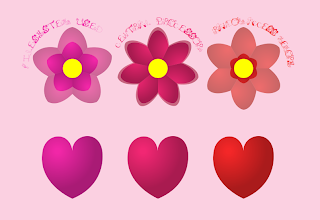Another, and most likely the last, variation on my valentine flower Lua script and a final entry in the conky of the month competition on the conky blog.

The biggest change here is the use of shading to the flower petals. Also I changed the shapes that were transformed by the output of the various conky objects so that there is no overlap. I think these changes have added quite a bit of eye-candy value to this conky and the effect was quite simple to apply.

The hearts were also drawn with cairo in the Lua script and shaded to match the flowers. Luckily I had a heart shape already on file from my first flower conky. I tweaked it a bit for shape, inspired by a wallpaper I found here.

I made an option to change the number of petals for each flower, but I didn't factor in how this would affect the shading. As a result changing the number of petals alters the shading effect. This should be easily fixed however if I feel like working on the script some more.

As ever, the code is posted on the crunchbanglinux forum here.

## Thursday, February 25, 2010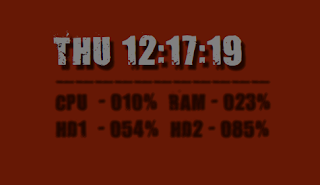Here is a simple script to add a blur like effect to text and numbers.
It can also be used to make shadows for text.

Here is a comparison of the blur effect in use and text without the effect.The effect works by taking multiple instances of the same text, making them mostly transparent and then overlaying them with a slight offset for each instance. In this case I am displaying the text at various points around a very small circle.

Like so: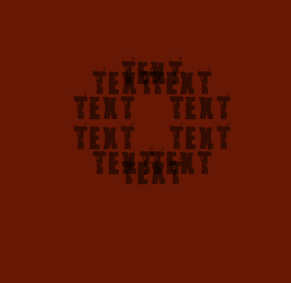The blur effect uses a much smaller circle and calculates transparency by the number of instances of the text you want.

You can get the code from my post on the crunchbanglinux forum here.

## Wednesday, February 24, 2010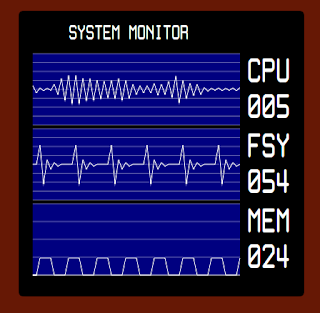I took my line graph Lua script, tweaked it a bit and produced the above.
The FSY "heartbeat" blip shows the filesystem used percent, then 1/4 of fsys used.

The MEM goes up to current memory use, stays there for two cycles then goes back to zero.

You can get the code here.

## Tuesday, February 23, 2010

I was having some trouble with getting the line graph working properly. In fact a few hours were spent getting frustrated with the lua script because it wasn't doing what I wanted it to do!

But then I had a breakthrough and after that it was pretty straightforward :)
This is what the current script is capable of displaying.In fact the first figure was what I had been after for a while. I wanted my graph to resemble an electroencephalogram.

I'm sure there is more that can be done from this basis so I may well be posting updates in the future.

You can get the code from my post in the crunchbanglinux forum here. You can also watch a short clip of an earlier form of this script in action.

The problem was the transfer of information from the data generating function to the figure drawing function. The problem was that you can only transfer data held in strings. In the data function I was generating a table something like this:

table={}
n=10
for i=1,n do
table[i]=i
end

This would give me a table with 10 entries, the entries being the numbers 1 to 10.
If i did this:

print (table[i])

I would get this output in the terminal:

1
2
3
4
5
6
7
8
9
10

Which has been fine so far, but the table I was using was much more complicated than this and I just couldn't get things working by simply transferring the string "table[i]" to the figure drawing function. What I really needed was to transfer the whole table to the drawing function.

The breakthrough was the use of this command:
string=table.concat(table, ":")

What this does is to take a table and turn it into a string, in this case, each entry in the table will be seperated from the next by a ":" in the string. So our example table from above, print (string) would output the following:
1:2:3:4:5:6:7:8:9:10

I could then take the string, pass it to the drawing function and use the split string function to turn it back into a table. Then I could work from the table to achieve the figure.
In response to a question asked about vertical graphs on the ubuntu conky thread, I thought that the script I had come up with for bar graphs could be easily modified to give vertical bars. And it was pretty easy.

Then I thought about how I could apply a gradient effect along the bar graph, like you can get in a regular conkyrc, and that wasn't too hard either. So now the script can output graphs like this:The code to do this has been posted on my original crunchbang linux forum post.

Alternate graphs.The standard graph outputs from conky are rather uninspiring, so thought I would try and put together some alternatives. The above screenshot is displaying cpu usage over time. Usage is displayed as both a negative and positive value at each point, so you get this kind of "waveform".

You can get the code on the crunchbanglinux forum here.

In the scrip there is a setting that can produce 3 types of graph, the up and down as above, only up or only down as below.Another improvement over the regular graphs is that you can change which direction the graph moves in.

Other configurables include:

• colors
• total height
• bar width
• spacing
• gridlines on or off

I am also working on a line graph, but have run into a few problems, and I havnt got it working how I want it yet. But it may not be possible to do what I want either :)
This is what it currently looks like:I think this graph has a lot of potential however!
See subsequent blog entries for developments.

## Monday, February 22, 2010

When to use "for i = do" and a look at drawing with cairo.

I was fiddling with my updated flowers conky, drawing a bumpy circle with cairo to use as the flowers center. Drawing with cairo via a text file isn't the most user friendly thing you can do, but with some experiementation I had worked out a good way to draw a repeating apttern in a cicle using the cairo_curve_to command.

The approach involved defining a set of circles, and then using the mathematics available in Lua to generate plot points.

Like so:
but first I need to set up some variables!
inner=110
outer1=120

outer2=118
outer3=120

The 4 lines above set out the radius of the circles from which I will be calculating my plot points.
varth1=0.53
varth2=0.42
The above 2 lines will be seen in action later.
hori=150
vert=200

These are positional setups.
Now I can do the drawing.

cairo_translate (cr, hori, vert)
cairo_move_to (cr, 0, 0)
I like to use the translate command for positioning figures. The reason for this is that it allows you to assign the figure "local" coordinates of 0,0 so that you can apply rotations to the figures without cauing problems. When using trandlate in a drawing function you always need to translate back at the bottom of the function. In this case I would translate back with:
"cairo_translate (cr, -hori, -vert)

--inner arc from cairo_set_source_rgba (cr, 1, 1, 1, 1);
text_arc=((2*math.pi/24)*(3))

txs1=0+inner*(math.sin(text_arc))
tys1=0-inner*(math.cos(text_arc))

Above I am setting a starting point for our drawing. The first line is calculating radians for points around the circle. In this case I have divided the circle up into 24 pieces and we are getting the radian value for point number 3. Theres no reason I started at 3, thats just how it happened.
I am also using comments to remind me whats what.

--outer arc through bump1
text_arc=((2*math.pi/24)*(3+varth1))
txs2=0+outer1*(math.sin(text_arc))

tys2=0-outer1*(math.cos(text_arc))
This is the "control" point that will determine the extent of the curve.
Take a look here to see how cairo works out its curves (scroll down a little for curves.)

--outer arc to
text_arc=((2*math.pi/24)*(4))

txs3=0+outer2*(math.sin(text_arc))

tys3=0-outer2*(math.cos(text_arc))
cairo_move_to (cr, txs1, tys1)

This is the coodinates of where the curve will finish.

cairo_curve_to (cr, txs1, tys1, txs2, tys2, txs3, tys3)
And above the curve is being drawn. Because I am drawing very simple curves (arcs really but I find the use of curve_to easier and more understandable than arc_to) I am basically getting this effect:So the above drawing made a curve out, now we will curve back in, from point txs3,tys3 to coordinates txs5,tys5 and that curve will be controlled by point txs4,tys4 as below.

--outer arc through
text_arc=((2*math.pi/24)*(4+varth2))
txs4=0+outer3*(math.sin(text_arc))
tys4=0-outer3*(math.cos(text_arc))
--outer arc to
text_arc=((2*math.pi/24)*(5))
txs5=0+inner*(math.sin(text_arc))
tys5=0-inner*(math.cos(text_arc))
cairo_curve_to (cr, txs3, tys3, txs4, tys4, txs5, tys5)

This gives me one bump like this:Then originally I did this:
--outer arc through bump2
text_arc=((2*math.pi/24)*(5+varth1))
txs6=0+outer1*(math.sin(text_arc))
tys6=0-outer1*(math.cos(text_arc))
--outer arc to
text_arc=((2*math.pi/24)*(6))
txs7=0+outer2*(math.sin(text_arc))
tys7=0-outer2*(math.cos(text_arc))
cairo_curve_to (cr, txs5, tys5, txs6, tys6, txs7, tys7)
--outer arc through
text_arc=((2*math.pi/24)*(6+varth2))
txs8=0+outer3*(math.sin(text_arc))
tys8=0-outer3*(math.cos(text_arc))
--outer arc to
text_arc=((2*math.pi/24)*(7))
txs9=0+inner*(math.sin(text_arc))
tys9=0-inner*(math.cos(text_arc))
cairo_curve_to (cr, txs7, tys7, txs8, tys8, txs9, tys9)
--outer arc through bump3
text_arc=((2*math.pi/24)*(7+varth1))
txs10=0+outer1*(math.sin(text_arc))
tys10=0-outer1*(math.cos(text_arc))
--outer arc to
text_arc=((2*math.pi/24)*(8))
txs11=0+outer2*(math.sin(text_arc))
tys11=0-outer2*(math.cos(text_arc))
cairo_curve_to (cr, txs9, tys9, txs10, tys10, txs11, tys11)
--outer arc through
text_arc=((2*math.pi/24)*(8+varth2))
txs12=0+outer3*(math.sin(text_arc))
tys12=0-outer3*(math.cos(text_arc))
--outer arc to
text_arc=((2*math.pi/24)*(9))
txs13=0+inner*(math.sin(text_arc))
tys13=0-inner*(math.cos(text_arc))
cairo_curve_to (cr, txs11, tys11, txs12, tys12, txs13, tys13)

etc etc...

Drawing the lines for the curves one by one, out then in, out then in... until I got all the way around, closed the path and filled it in. This was was pretty easy, just copy and paste and edit the numbers. I also pretty much knew that I didn't have to number the strings in order but I did it anyway. For example:
string=1
print (string) --> 1
string=2
print (string) -->2

But I could see that there was an obvious pattern and was a candidate for using the:

"for i = x,y do" command.

So I looked at the first bump I had drawn, assigned i a value of 1 and then edited the values so that the points I was plotting on the circles were expressed via i, point 3 being i+2 and point 4 being i+3. And setting up a string to supply the number of points, substituting the string into the code. What I ended up with is this:

number=12
for i = 1,number do
cairo_set_source_rgba (cr, 1, 1, 1, 1);
--inner arc from
text_arc=((2*math.pi/number)*(i+2))
txs1=0+inner*(math.sin(text_arc))
tys1=0-inner*(math.cos(text_arc))
--outer arc through bump1
text_arc=((2*math.pi/number)*((i+2)+varth1))
txs2=0+outer1*(math.sin(text_arc))
tys2=0-outer1*(math.cos(text_arc))
--outer arc to
text_arc=((2*math.pi/number)*(i+3))
txs3=0+outer2*(math.sin(text_arc))
tys3=0-outer2*(math.cos(text_arc))
cairo_move_to (cr, 0, 0)
cairo_line_to (cr, txs1, tys1)
cairo_curve_to (cr, txs1, tys1, txs2, tys2, txs3, tys3)
--outer arc through
text_arc=((2*math.pi/number)*((i+3)+varth2))
txs4=0+outer3*(math.sin(text_arc))
tys4=0-outer3*(math.cos(text_arc))
--outer arc to
text_arc=((2*math.pi/number)*(i+4))
txs5=0+inner*(math.sin(text_arc))
tys5=0-inner*(math.cos(text_arc))
cairo_curve_to (cr, txs3, tys3, txs4, tys4, txs5, tys5)
end
cairo_close_path (cr)
cairo_fill (cr)

But it wasn't quite so simple. For some reason the shapes of the bumps changed (they were alot more pointy instead of round) and I had to add these lines or the middle of my drawing did not get filled in:
cairo_move_to (cr, 0, 0)
cairo_line_to (cr, txs1, tys1)

But it was quite easy to fiddle with the settings to make the bumps rounded and voila!.

So when you have a repeating pattern, of you want multiple instances of a figure, then it's a good bet that you can cut down the line count by using this technique. You can also affect other things with the "i" term. Achieving a position change from figure to figure you could do something like:

horizontal = 10*i
vertical = 10

in this case you would get a horizontal line of figures, spaced 10 apart.

But you could also do something like:
cairo_set_source_rgba (cr, 1, 1, 1, 0+(i/10)

So if "i" has a range of 1 to 10, the first figure would have an alpha of 0.1 while the tenth figure would have an alpha of 1.

Or you can fit "i" into any number of equations and have it affect any number of variables.

And if you want more figures, just increase the range of "i" without having to add any additional lines of code.

## Friday, February 19, 2010Three Dimensional Bars! Well, I guess to be more accurate they would be called isometric?
I had been thinking about doing this for a while and it seemed like an obvious idea :)

Anyway, I thought this project would be easy, and to begin with it was. Getting a bar on Lua is pretty easy, then all I would have to do is draw a top and a side and get everything moving correctly. This all happened quickly until I started to look at the lines!

Unfortunately I couldn't simply take the shapes I had drawn to form the "faces" with fill, and overlay them using stroke. The corners stuck out when the line width was increased and the different line unison options in cairo were not going to work. So I had to re-do the lines from scratch, and it took far too may attempts and corrections before I finally got it right.

With this script you can specify:
• face color
• line color
• bar width ("depth" is always set to width/2)
• bar height
• bar position
• wire-frame outline on or off
• and font, font size, font color and font position
You can get the code on the crunchbanglinux forum here.

## Thursday, February 18, 2010More ring meter variations.
I have added the ability to draw:
• anticlockwise indicator
• rounded ends to indicator lines
• rounded end borders
• and square end borders
You can get the cod on the crunchbanglinux forum here.

## Wednesday, February 17, 2010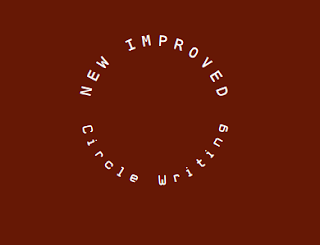I have improved my circlewriting function significantly! No more annoying fiddling about to get it right, the function puts everything in the right place first time. I have kept the option to fine tune through variables to take into account the variations in fonts. I find this function works best with a monospace font anyway.

There are 2 function:
"circlewriting" writes text across the top of a circle, on the outside.
"circlewritingdown" writes text along the bottom of a circle on the inside.
Like so:You can get the code for these functions here on the crunchbanglinux forum.

Several of my previous Lua scripts have used predecessors of this function. For those, just delete the old function, put in the new functions and make sure that when you activate the function you are giving it all the right information. In the code in the link above I have made the way that you feed the function data a little easier:

--text must be in quotes
text=" Circle Writing "
--font name must be in quotes
font="White Rabbit"
fontsize=18
positionx=100
positiony=150
colorred=1
colorgreen=1
colorblue=1
coloralpha=1
letterposition=0
letterrotation=0
circlewritingdown(cr, text, font, fontsize, radius, positionx, positiony, colorred, colorgreen, colorblue, coloralpha, letterposition, letterrotation)

Information is entered line by line with well described strings.

You can also use this kind of display for "live" information. Here I am using the addzero100 function so that the numbers are always 3 digits, although because now the "text" string length is read automatically, the display will alter itself with altering length of "text".Here is the text strings that achieved the above:

cpu=tonumber(conky_parse('\${cpu}'))
text=(" CPU " .. (addzero100(cpu)) .. "% ")

mem=tonumber(conky_parse('\${memperc}'))
text=(" MEMORY " .. (addzero100(mem)) .. "% ")

and for reminder here is the addzero100 function:

if tonumber(num) < 10 then
return "00" .. num
elseif tonumber(num) <100 then
return "0" .. num
else return num
end
end

I have now included the ability to set a start and finish position for the displayed text so that you can achieve results like this:I have updated my post on the crunchbanglinux forum here. The code there outputs the above image. There is also a brief discussion regarding overcoming the alignment problems that are inherent in this function.
ANOTHER UPDATE :)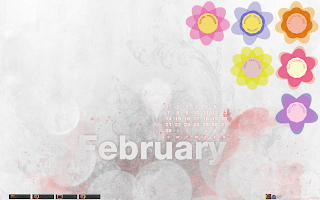I was looking at the code I had written and the cairo commands to draw the flower shapes clearly had repeating patterns, so I decided to use the old "for i =" trick to make the code smaller. This worked and I managed to cut down the line count quite considerably. Then I realized that having done that I could change the range that i is equal to. The end result is that you can now specify the number of petals for each flower, and the bumpy middle always has 2x the number of petals.Conky flowers revisited.
Go here to the crunchbang forum for the latest code.

UPDATE - I have updated the code in the post above so that the Lua script has my improved circlewriting function.
UPDATE2 - I wasn't completely happy with the circular middles, they seemed too hard. So I have enabled the option to draw bumpy middles as so:Some significant changes and some significant improvements.
First the improvements:
• flower are placeable independently
• flowers can be rotated in place without anything else being effected
• flowers can be used with any wallpaper and allow transparency effects and overlap

The third improvement was accomplished by redesigning the flower's color change mechanism. In the original the flower color change "effect" was created by placing a circle that changed size in response to a numerical input behind a flower "cutout" (see my crunchbang post to see what I'm talking about). But with the revisit, each petal moves independently from each other (except they are all fed the same number although it would be possible to have each petal represent a separate data output). Everything is contained within the flower shape.

There are some things I need to work on. The circlewriting function needs work. I think I know how to get it working better, but right now it is far too fiddly, adjusting the "variables" to make everything look right.

Anyway, this is yet another (or an alternative) entry in the conky blog valentine competition.

## Monday, February 15, 2010Here is another Lua script. For the code and to see it in action go here to my post on the crunchbang forum.

require 'cairo'

I have describe the below function in my full screen lua script. This function is here because at first I was just trying to generate numbers arranged around a circle, and I wanted all the numbers to be 3 digits constantly. When I first wrote the function It was to output numbers around a circle. I suppose I need to re-write this script for the sake of clarity :)
if tonumber(num) <>
return "00" .. num

return "0" .. num

else
return num
end

end

The below function is the figure drawing function. This was taken from the circle-writing function I wrote for my valentines flower conky.

function circlewriting(inum, text, ival, font, fsize, radi, horiz, verti, tred, tgreen, tblue, var1, var2)
Above is the function name followed by a long list of strings that the function needs to work.

inum - "length" ie the number of instances that the data will move to. In the above image this number was 10.

text - the actual data that is being represented, in this case cpu usage.

ival - in the below function an array is used to generate the data and in that array we will be using the code line:
for i = 1, tonumber(len_t) do
len_t is the same number as "inum" above. So "i" is all the numbers between 1 and 10.

font - to set the font for the text output
fsize - sets the font size
radi - sets the radius of the circle around which the bars or text will be displayed.
horiz - horizontal, x, position.
verti - vertical, y, position
tred - output red color value
tgreen - output green color value
tblue - oputput blue color value
var1 - variable 1
var2 - variable 2

variables 1 and 2 are used to fine tune the position and orientation of the bars or text around the circle.

deg=360/inum
Above is a quick calculation to work out how many degrees there are between each bar. This will be used for the rotation of each bar.

Now comes the hard math!
text_arc=((2*math.pi/inum)*ival+var1)
The above 3 lines are equations to work out the positions of each bar around the circle.
As we all know: circumference = 2 x pi x radius
and to calculate coordinates for each point around a circle
and

Where "n" is the number order of the thing that is being displayed. For example, on a clock face there are the numbers 1 to 12. So to get the x,y position of those numbers, n would be 0 for 12, 1 for 1, 2 for 2 etc.

But Lua only works with radians so the first line is converting degrees to radians.
The next 2 calculate the x and y plot points.

cairo_select_font_face (cr, font, CAIRO_FONT_SLANT_NORMAL,
CAIRO_FONT_WEIGHT_NORMAL);

cairo_set_font_size (cr, 22);
cairo_set_source_rgba (cr, tred, tgreen, tblue, ival/10);
The above lines are setting the font, font size and color.

Below are the lines that generate the bars. I worked out how to apply the rotation to the bars "locally" and position them with the translate command.

First the position is translated into position.
cairo_translate (cr, txs+horiz, tys+verti)
Then the bar is rotated below. You might wonder why I am taking the "deg" string, set earlier, and performing calculations on it to convert degrees into radians, when I have already done that 8 lines earlier when I was calculating the x,y plot points of my bars. The answer is simple... when I first wrote the function for the flowers.lua my math skills re circles were extremely rusty, so instead of trying to work out the x,y equations for myself, I borrowed then from dissecting londonali1010's air clock lua script (found here) without really understanding the math but knowing it worked. So when I implemented the rotation feature I wrote the calculations seperately. A case of historical artifacts. Just another thing to clean up in this script :)
cairo_rotate (cr, (deg*(ival+var2)*(math.pi/180)))
Then the rectangle is drawn at "local" position 0,0.
cairo_rectangle (cr, 0, 0, 20, (text*-1))
cairo_fill (cr)

Then the rotation is reset.
cairo_rotate (cr, ((deg*(ival+var2)*(math.pi/180)*-1)))
And finally the translation point is reset. Remember that all cairo transformations are cumulative so if these reset steps were not done then the next bar would be translated and rotated relative to the first bars position and rotation.
cairo_translate (cr, -1*(txs+horiz), -1*(tys+verti))

Here are the lines that generate the text output. I decided to comment them out as I liked the lookof the bars better. But they can be reinstated easily
--you can reinstate these lines to get a text output around the circle
--cairo_move_to (cr, txs+horiz, tys+verti);
--cairo_rotate (cr, (deg*(ival+var2)*(math.pi/180)))
--cairo_rotate (cr, ((deg*(ival+var2)*(math.pi/180)*-1)))
end
end
Thats the end of the figure drawing function.
Next comes the data generating function.

function conky_draw_graph()
Above is the name of the function, this is the function we will call in conky.
if conky_window == nil then return end
local cs = cairo_xlib_surface_create(conky_window.display, conky_window.drawable, conky_window.visual, conky_window.width, conky_window.height)
cr = cairo_create(cs)
Above is the "canvas" setup just set out a little differently from how I have done it before.

len_t=10
t1={}
end
The above lines are particularly important. Here we are initializing the variable. Basically we are telling Lua that the string called "t1" is a table. We are also setting the "length" of the figure with len_t. It took me a little figuring out to understand why these lines had to be here and why they were set only when conky update number = 1.
The reason is that if the line "t1={}" were moved below with the rest of the function (which has to start when conky updates >3 to prevent a segmentation fault when using the conky object \${cpu}) then with each cycle, the line:
would be true and everything below that line would be performed and the data stored table "t" would be reset each time. We are relying on the data in the table to "remember" the past cpu usage numbers so t1={} can only be set once.
Below is the array that will generate the data and store it in the table.
And here is the all important "i"
for i = 1, tonumber(len_t) do
Here we are saying that "i" is equal to every number from 1 to len_t (set above). So to think about this array more simply we will say that "i"=1
if t1[i+1]==nil then t1[i+1]=0 end
The above line is a error checking line to stop the "tried to call a nil value" error that can cause the script to stop functioning.
t1[i]=t1[i+1]
So when "i"=1 then
t1=t1.
As we have seen before, numbers, or strings in square brackets relate to an entry in a table, in this case table "t1". This doesnt make much sense until we get to the next couple of lines:
if i==len_t then
t1[len_t]=tonumber(conky_parse('\${cpu}'))
end
So when "i" = the upper limit of its range, len_t (which in this case is 10) then t1=current cpu usage. Now everything else is set because
t1=t1
t1=t1
t1=t1
to
t1=t1

Now you might think that if everything equals everything else then why isn't t1 to t1 all the same number? What you need to think about here is how the Lua script is working with conky. Say you have your conkyrc set to update every second, then every second the Lua script is run from the top, so actually the example I gave above about how the table is set up is the wrong way round.

Because the script lines are performed in order from top to bottom here is what is happening: (or this is how it makes sense to me)

on the first pass at time 1:
t1=cpu usage at time 1

then on the next pass at time 2
t1=t1 *but* this is the t1 from the previous pass where t1=cpu at time 1
because t1 is set every so slightly before t1 is reset to equal cpu usage at time 2

and so on it goes so that
t1 = current cpu usage at time t
t1 = cpu usage at t-1
t1 = cpu usage at t-2
t1 = cpu usage at t-3
etc...

--circlewriting (inum, text, ival, font, fsize, radi, horiz, verti, tred, tgreen, tblue, var1, var2)
Above I am reminding myself of all the variable i need to send the circlewriting function and below I have called the function with all of the relevant info.
circlewriting(len_t, t1[i], i, "Mono", 22, 40, 200, 200, 0, 0, 0, -0.25, 0)
Below we are ending the part of the function that began "if updates> 3 then" It is important to put the call to the figure writing function above this end.
end
Below we are freeing some memory, althoug in action I havnt noticed any difference from when I use these lines to when I do not use these lines.
cairo_destroy(cr)
cairo_surface_destroy(cs)
end
And then we close the function.
end
I will probably revisit this explanation again soon to go over some parts more thoroughly.

## Sunday, February 14, 2010

This approach has the advantage of being much more compact and using less functions. I will be feeding the variables straight into the drawing function. The lua is to create bars and circle charts like so.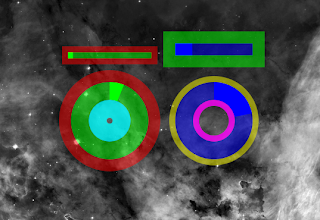You can get the whole code here on the crunchbang forum.

Here is the code lines in question:
The first line of the function that draws the bars is:
function draw_bar(co, width, height, across, down, bgr, bgg, bgb, bga, inr, ing, inb, ina, lw, lr, lg, lb, la, rotate)
you can see the name of the function followed by a long list of strings. These are all the strings that will be set later when the function is called. Here is a description of each string:

co = conky object data to display
width = how wide the rectangle will be
height = how tall the rectangle will be
across = x position
down = y position
bgr = red component of background color
bgg =green component of background color
bgb = blue component of background color
bga = alpha component of background color
inr = red component of indicator color
ing = green component of indicator color
inb = blue component of indicator color
ina = alpha component of indicator color
lw = width of the boundary line
lr = red component of line color
lg = green component of line color
lb = blue component of line color
la = alpha component of line color
rotate = rotation in degrees that you want applied to the bar

pretty straightforward variables, and I tried to make it so that you could more or less work out what the string names meant. Now here is the line when the function is called:
draw_bar(cpu, 150, 20, 75, 50, 0, 1, 0, 0.5, 0, 1, 0, 1, 10, 1, 0, 0, 0.5, 0)
Each value is passed up to the draw_bar function.
So that:
co=cpu
width=150
length=20
across=75
down=50
...etc etc.

Then you can call the same function again but feed it a different set of information like so:
draw_bar(mem, 150, 40, 250, 30, 0, 0, 1, 0.5, 0, 0, 1, 1, 20, 0, 1, 0, 0.5, 0)

Not as user friendly as the settings table approach but far less code and alot less complicated. Another important aspect to this approach is that it is more like a "tool" that can be used in a Lua script that contains multiple elements. All you need is the figure drawing function above the function that you will be calling in conky, then if you want a bar or circle meter in your setup, call the function with a single line setup.
I knew it could be done more easily.
Thanks to the coding skills of wlourf, the code to generate the moving bars/moving dots lua (here) is now significantly shorter! Here is wlourf first post on the crunchbang forum.

I knew it was possible and I knew it would involve the technique that wlourf used. I just haven't gotten to grips with the workings of arrays!

And here is a simplified code that generates the moving dot conky as I did before:

require 'cairo'
function dotdraw(cr, num, inum, length, hori, vert, width, height, dotsize, dotr, dotg, dotb, dota)
cairo_set_source_rgba (cr, dotr, dotg, dotb, dota)
modnum=(num*(height/100))
cairo_arc(cr, ((width*inum)+hori), (vert-modnum), dotsize, 0,2*math.pi)
cairo_close_path(cr)
cairo_fill (cr)
end

function conky_draw_graph()
if conky_window == nil then return end
local cs = cairo_xlib_surface_create(conky_window.display, conky_window.drawable, conky_window.visual, conky_window.width, conky_window.height)
cr = cairo_create(cs)
len_t=20
t1={}
end
for i = 1, tonumber(len_t) do
if t1[i+1]==nil then t1[i+1]=0 end
t1[i]=t1[i+1]
if i==len_t then
t1[len_t]=tonumber(conky_parse('\${cpu}'))
end
--dotdraw(cr, num, inum, length, hori, vert, width, height, dotsize, dotr, dotg, dotb, dota)
dotdraw(cr, t1[i], i, len_t, 10, 200, 10, 200, 3, 0, 0, 0, 1)
end
cairo_destroy(cr)
cairo_surface_destroy(cs)
end
end

Just a few hundred lines shorter! And using the array you can have a graph as long as you want without increasing the size of the code. All you have to do is change a variable.

Also important, in addition to the use of an array to generate the data, is that the graph drawing function (dotdraw at the top) is separated from the data generating function (conky_draw_graph). This means that the data can be fed easily into as many different kinds of drawing functions as you can think of to represent it. I can think of a number of ways of presenting "graph" like data and now all i have to do is create the drawing function and feed it the data.

In addition this method seemed ideal to apply to creating a text scroll function.
Here is the result:The code and a video clip are posted on the crunchbang forum here (it is still a work in progress).
I have never liked the native scroll function in conky because it is not continuous. If you have a scroll length of 100 say, then it will scroll your message but then follow it up with 100 empty spaces. Unfortunately this lua scroll has its drawbacks too. Principally that the information is static as it moves across, cpu usage will not change and time will not update, until the strings are generated for the next pass.

However, I think this scroll would be ideal for such things as time and date and to display the titles of music tracks.

I'm certainly going to be seeing what interesting formats I can come up with!

## Thursday, February 11, 2010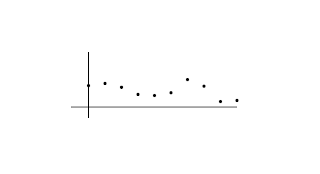These two graphs both display cpu usage over time. The blue graph came first and then I modified the Lua script to generate the dot chart. I had been thinking of tackling an animated bar chart for a while and decided that I should just give it a go. But it wasn't easy!

Just to conceptualize how it was going to work in the beginning took a while...
I had to think of a way to capture cpu usage information at one particular time, store that information and display it somehow while capturing new information every cycle and linking that all together to get the illusion of a moving chart.

I had (with the help of the crunchbanglinux forum) found a way to implement a timer in lua:

local timer=(update_num % 4)+1

Parse conky to get the updates number then using the line above, every time the updates number is divisible cleanly by 4 (ie no remainder) then timer =1 (without the +1 it would equal 0)
The result is that the timer counts 1,2,3,4,1,2,3,4,1,2,3,4 etc etc. This was the basis for the animation.

I started with trying a 4 bar graph and after a little figuring out I got to the point where i could get the bars set and moving across, but when the timer reset so did the bars. The problem was that I could pass information down the script by referencing strings:
num1=number
num2=num1
num3=num2
num4=num3
But to get the table working i needed this:
A->B->C->D->a->b->c->d
B->C->D->a->b->c->d->A
C->D->a->b->c->d->A->B
D->a->b->c->d->A->B->C

So that I get a full cycle, so that the next step I can reset back top the beginning. But going from dABC which existed at the bottom of the script back to the top for ABCD, I had to take the information for ABC (from dABC) and move it up the script so that ABC in ABCD is the same ABC from dABC. That makes sense right?

So I needed a function. Functions are how you move information from the bottom of the script to the top!

Anyway. Look here at the code on the crunchbang forum and you will see what I ended up with. The script is pretty long. The reason for this is that, as you can see from the above example, for a 4 step animation you actually need 8 steps, each step containing 4 bits of information. So for 4 steps you need 32 bits of information.

I ended up with a 10 step graph which needed 200 bits of information. If i wanted a 20 step graph then that is 20x2x20=800 bits of info.

If anyone has an easier way then I would be only too glad to hear it!

The other drawback with the graph drawing function, as I have written it, is that you can only feed it one set of information. Unlike other functions where you can feed it say cpu info and settings and also feed it memperc information and settings and get 2 outputs. This function you cant do that...something just doesn't work. It would be easy enough to copy the function, and change its name, then call the newly named function with different information in the conky display function. However, it is also the case that there arent many other conky outputs that are suited to a graph.

Downspeed and upspeed graphs can be generated by conky, and could be generated from my graph drawing script... but extra steps would need to be taken to adjust for the changing units... B to KiB to MiB.

Maybe I'll find a more compact way of doing this... although running this script, total "resting" cpu usage on my system is around the 2's or 3's so it is hardly intensive even for it's size.

## Wednesday, February 10, 2010

Hot on the heels of my custom bars lua comes another script for drawing bars and rings. And after figuring out most of what goes into making a settings table work with cairo in a lua script... I decided to go with another approach.

Instead of setting up a settings table at the beginning of the script, then parsing the table with one function and feeding the parsed information into another function which converts the table values into local strings... I went with defining the values when calling the draw function. It maybe isn't as user friendly as the settings table way but in less than half the lines of my custom bars lua I have created a script that can generate the same bars and also generate ring meters.

Also I think the way that I have set up the code will make it easier to inset these meters into another Lua script. All it requires is that the meter drawing functions are placed above the function to be called in conky. Then you can use the functions with just a couple of setup lines.

Of course the ring meters are taken from londonali1010's ring meter script here. Many thanks to londonali1010 for all her excellent and inspirational work!I have also enabled the addition of boundary rings for the ring meters. The outer and inner boundary rings can be set values for width, color and alpha individually.

The code in posted in the crunchbanglinux forum conky config thread here.
I have just updated the code to allow much easier use of rotation with the bars. Bars rotate in place and not relative to 0,0 in the new code.
Here is the annotated Lua script for my custom bars.For simplicity I have put in the settings for only 1 bar.

require "cairo"

So first we have the table below.
The table has a format like this:
table={{A1=1,B1=2,C1=3,D1=4},{A2=1,B2=2,C2=3,D2=4},{A3=1,B3=2,C3=3,D3=4}}
but for ease of use and so that the settings can be edited easily the table is split up line by line as follows:
BUT you still have to have all of the brackets (I'm not sure that they have to be curly brackets, but they certainly work.) and all of the commas or you will get an error.
table = {
{
I have used comments throughout the table so that each setting is easily identified. The comments do not interrupt the format of the table.
--conky object for output
co='cpu',
--rectangle width
rw=150,
--rectangle height
rh=50,
--NOTE about width and height. For a bordered rectangle with line width lw (specified below)
--the border is drawn 1/2 lw inside and 1/2 lw outside the boundary of the rectangle, so that
--final width=rw+lw and final height=rh+lw. I did not automate this below as it is possible
--to specify a line width (lw) greater than either width (rw) or height (rh).
--eg if you want a rectangle 200 pixels long and a border line width of 8, then rw=100-8=92
Above is a note about how to set up the heights and widths. If something isn't obvious to anyone who might use the script it's nice to explain things,
--background rectangle color set
bg_red=1,
bg_green=0,
bg_blue=0,
--background rectangle alpha
rab=0.5,
--indicator rectangle color set
in_red=0,
in_green=1,
in_blue=0,
--indicator rectangle alpha
rai=1,
--border line width
lw=40,
--border line color set
l_red=1,
l_green=0,
l_blue=1,
--border line alpha
la=0.2,
--position, x and y
rx=200,
ry=150,
--set rotation in degrees (will require x and y to be reconfigured)
rot=0
},
}
So we have all of the variables set. Each variable will be passed into the various functions and applied to the bars when they are drawn.

There are 2 functions and one sub function in this script. From looking at my last annotated script a function is written and then called below, and then when it is called information is passed up to the function. Here, to see how the information is passed I'll look at the second function first. This is the function that we will be calling in conky:
function conky_draw_shape()
this is the name of the function
if update_num > 5 then
if conky_window==nil then return end
local w=conky_window.width
local h=conky_window.height
local cs=cairo_xlib_surface_create(conky_window.display, conky_window.drawable, conky_window.visual, w, h)
cr=cairo_create(cs)
The above should be familiar. It sets the 5 cycle delay and the surface onto which cairo will draw. Next comes a local function. This function called "parse" which takes the table entry "co" (the conky object whose output will be displayed by the bar) and performs the conky_parse command on it.
local function parse(cr,pt)
Here is the local function name and the strings that will be set when the function is used below.
local str=''
local value=0
str=string.format('\${%s}',pt['co'])
The pt part is the important part (note that there is nothing important about the term pt, it is just the name of a string and could be anything. Perhaps pt stands for parse table). pt is one of the strings that is set by the parse function from information fed to the function when it is called. Also you should remember 'co' as one of the variables we set in the settings table.
str=conky_parse(str)
value=tonumber(str)
The function is parsing 'co' the conky object, running that through the tonumber command and then storing the information in a string named "value"
if value == nil then value = 0 end
draw_table(cr,pt,value)
The line above is calling the first function in the script, and feeding the function 3 things: cr, pt and value. As we saw the "value" string is generated above and it is that string that will be passed to the draw_table function.
end
Here is the end of the local function. Below is the remaining commands of the "global" function called "draw_shape"
for i in pairs(table) do

parse(cr, table[i])
These 2 lines is where the "magic" happens :) These lines read the contents of the table, and pass each set of variables to the parse function. In this case the letter "i" is extremely important and cannot be substituted. Perhaps "i" stands for "instance" or "index", I don't know exactly. So basically what is being done is that for every set of data ie {A1=1,B1=2,C1=3,D1=4} in the settings table the "parse" function is being called and fed the cr string as well as every string that the set contains ( A1, B1, C1, D1).

As you can see the parse function is being sent 2 things cr and table[i]. Then as we saw the parse function (local function parse(cr,pt)) takes cr and stores it as cr and takes the output of table[i] and stores it as pt. Then in the parse function we see pt['co'] which is the same as "table['co']". So the table is being searched for the string co, and the value of co is being formatted (str=string.format('\${%s}',pt['co'])) and stored in the string called "str".

So for the data set "table= {A1=1,B1=2,C1=3,D1=4}"
table['A1']=1
table['B1']=2
table['C1']=3
table['D1']=4

and as in the parse function table[i] = pt[i] so that

table['A1']=1=pt['A1']
table['B1']=2=pt['B1']
table['C1']=3=pt['C1']
table['D1']=4=pt['D1']

I hope that all makes sense.
So to recap...
• function draw_shape is generating the strings: cr and table[i]
• function parse is being fed cr (but not doing anything with it) and table[i] and renaming table[i] as the string pt.
• parse is looking through the string "pt" for the table setting 'co' then parsing and formatting the output and storing it as the string "value".
parse is then feeding the strings "cr", "pt" and the new string "value" up to the function settings_table.
end
end
end

Now we are going back to the point after the settings table (confused yet? :) )
Here we have the first function in the Lua script and we have already seen where the strings cr, pt and value are being fed from.
function draw_table(cr, pt, value)
local width=pt['rw']
local height=pt['rh']
local bgalpha=pt['rab']
local indalpha=pt['rai']
local across=pt['rx']
local down=pt['ry']
local lwide=pt['lw']
local lalpha=pt['la']
local bgcolr=pt['bg_red']
local bgcolg=pt['bg_green']
local bgcolb=pt['bg_blue']
local incolr=pt['in_red']
local incolg=pt['in_green']
local incolb=pt['in_blue']
local lcolr=pt['l_red']
local lcolg=pt['l_green']
local lcolb=pt['l_blue']
local rotate=pt['rot']
Above are a number of lines that are searching the table information (stored in the string pt) for the various bits and pieces of information. Then when the specific bit of information is found, that information is being set as a local string. For example:
local width=pt['rw']
string pt is being searched for the table setting "rw" (rectangle width) and storing the value of this setting in the local string "width".
--indicator calculation
local inum=(((width-lwide)/100)*value)
The above calculation allows the bars to be resized while maintainig the correct proportions of the indicator line.
--set initial rotation
cairo_rotate (cr, rotate*math.pi/180)
Here we are applying the local string rotate (initially set in the settings table as "rot") and performing the rotation, if any.
--background bar
cairo_rectangle (cr, (across+(lwide/2)), (down+(lwide/2)), (width-lwide), (height-lwide))
cairo_set_source_rgba (cr, bgcolr, bgcolg, bgcolb, bgalpha);
cairo_fill (cr)
Above we are drawing the background bar using the local strings we set above. The calculations in the code are to account for the way that the outline of rhe rectangle is drawn. This was explained towards the top of the script.
--indicator bar
cairo_rectangle (cr, (across+(lwide/2)), (down+(lwide/2)), inum, (height-lwide))
cairo_set_source_rgba (cr, incolr, incolg, incolb, indalpha);
cairo_fill (cr)
Above we generate the indicator bar.
--border line
cairo_set_line_width (cr, lwide);
cairo_rectangle (cr, across, down, width, height)
cairo_set_source_rgba (cr, lcolr, lcolg, lcolb, lalpha);
cairo_stroke (cr)
And above we draw the outline, if any.
--resets rotation
cairo_rotate (cr, (rotate*-1)*math.pi/180)
Then we reset rotation.
end
Below you can look through the functions that have been described above, but in the correct order.
function conky_draw_shape()
if update_num > 5 then
if conky_window==nil then return end
local w=conky_window.width
local h=conky_window.height
local cs=cairo_xlib_surface_create(conky_window.display, conky_window.drawable, conky_window.visual, w, h)
cr=cairo_create(cs)
local function parse(cr,pt)
local str=''
local value=0
str=string.format('\${%s}',pt['co'])
str=conky_parse(str)
value=tonumber(str)
if value == nil then value = 0 end
draw_table(cr,pt,value)
end
for i in pairs(table) do
parse(cr, table[i])
end
end
end
That's the end.
Here is an approach that achieves the same result as a settings table, in that you can achieve multiple outputs from a single instance of a function. But I think that this next approach is easier to implement and considerable shorter code wise. And here is my first take at explaining the use of arrays in a Lua script.